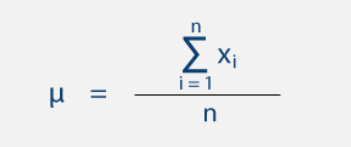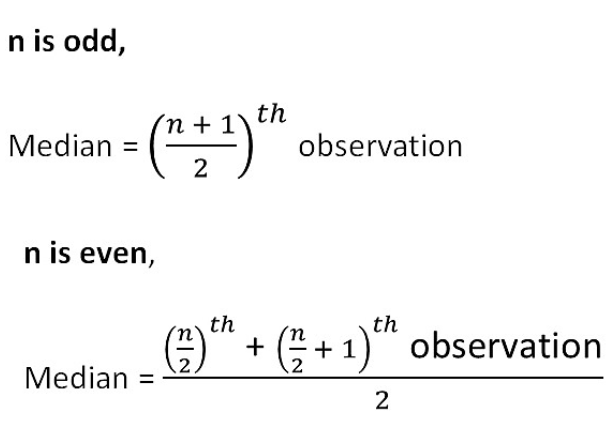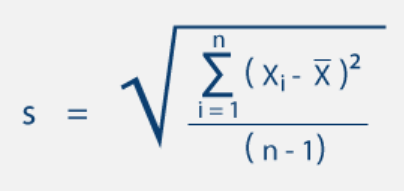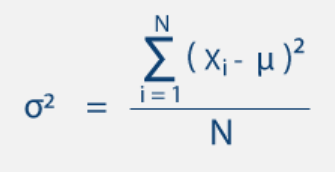# Statistical Computing using NumPy

## Introduction

The field of statistics is concerned with gathering and interpreting data. It includes methods for obtaining samples, describing data, and then concluding that data. NumPy is the core package for scientific calculations (refer to this blog for basics about NumPy). Hence NumPy statistical Functions go hand-in-hand with it.

NumPy has several statistical functions that can be used to analyze statistical data. When looking for a maximum or minimum of elements, these statistical functions come in handy. It's also utilized to figure out basic statistical notions like standard deviation and variance, among other things.

## Statistical Functions in NumPy

1. np.amin() - This function finds the element's minimal value along a specified axis.
2. np.amax() - This function determines the element's maximum value along a specified axis.
3. np.mean() - It determines the data set's mean value.
4. np.median() - It determines the data set's median value.
5. np.std() - It is used to calculate the standard deviation.
6. np.var() - It is used to calculate the variance.
7. np.ptp() - It gives you a range of values along an axis.
8. np.average() - It is used to calculate the weighted average.
9. np.percentile() - It calculates the data's nth percentile along the provided axis.

## Finding maximum and minimum of the array in NumPy

The NumPy functions np.amin() and np.amax() can be used to get the minimum and maximum values of array members along a specified axis.

``````import numpy as np

arr = np.array([10, 12, 14, 100, 3, 50])
print(arr)

# Numpy Minimum function
_min = np.amin(arr)
print(_min)

# Numpy Maximum function
_max = np.amax(arr)
print(_max)``````

Output

``````[ 10  12  14 100   3  50]
3
100``````

## Mean, Median, Standard Deviation and Variance in NumPy

### Mean

The mean is the sum of the elements divided by their sum, as calculated by the formula below.(source)

The axis along which the mean can be determined can also be mentioned.

``````import numpy as np

arr = np.array([10, 11, 12])
print(arr)

# Numpy Mean function
_mean = np.mean(arr)``````

Output

``````[10 11 12]
11.0``````

### Median

The array's median is the element in the middle. For odd and even sets, the formula is different.(source)

For both one-dimensional and multi-dimensional arrays, it can calculate the median. The median distinguishes between the upper and lower ranges of data values.

``````import numpy as np
arrOdd = np.array([10, 11, 12])
arrEven = np.array([10, 11, 12, 13])

# Numpy Median function
# for odd length
_median = np.median(arrOdd)
print("For Odd length : ",_median)

# for even length
_median = np.median(arrEven)
print("For Even length : ",_median)``````

Output

``````For Odd length :  11.0
For Even length :  11.5``````

### Standard Deviation

The square root of the average of square deviations from the mean is the standard deviation. The standard deviation formula is as follows:(source)

``````import numpy as np
arr = np.array([10, 11, 12])

# Numpy Standard Deviation function
_std = np.std(arr)
print("Std. Dev : ",_std)``````

Output

``Std. Dev :  0.816496580927726``

### Variance

The average of the square deviations is the variance. The formula for this is as follows:(source)

``````import numpy as np
arr = np.array([10, 11, 12])

# Numpy Variance function
_var = np.var(arr)
print("Variance : ",_var)``````

Output

``Variance :  0.6666666666666666``

## Average function in NumPy

The np.average() method in NumPy calculates the weighted average along with multi-dimensional arrays. The component's weight is multiplied by its weight to obtain the weighted average; the weights are supplied individually. If no weights are supplied, the result is the same as the mean.

``````import numpy as np
arr = np.array([10, 11, 12])

# Numpy Average function
#without weight same as mean
_avg = np.average(arr)
print("avg : ",_avg)

#with weight gives weighted average
wt = np.array([8,2,3])
_weightedAvg = np.average(arr, weights=wt)
print("weighted avg : ",_weightedAvg)``````

Output

``````avg :  11.0
weighted avg :  10.615384615384615``````

## Percentile function in NumPy

A percentile is a statistician's term for the value below which a given percentage of observations in a collection of data falls.

``````import numpy as np
arr = np.array([10, 11, 12])

# Numpy Percentile function
_percentile = np.percentile(arr, 20, 0)
print("Percentile : ", _percentile)``````

Output

``Percentile :  10.4``

## Peak-to-Peak function in NumPy

The np.ptp() NumPy method can be used to find the range of values along an axis.

``````import numpy as np
arr = np.array([[10, 11, 12], [20, 20, 20]])

# Numpy Peak-to-Peak function
_peakToPeak = np.ptp(arr, 0)
print("peakToPeak : ", _peakToPeak)``````

Output

``peakToPeak :  [10  9  8]``

## FAQs

1. What is percentile and how to calculate it using NumPy?
A percentile, in the most common sense, is a number below which a specific percentage of scores fall. Percentile can be calculated using the percentile() function in Numpy.

2. Explain the measures of central tendency in Python.
Measures of central tendency are mean, mode, and median. NumPy has built-in functions for calculating each of these.

3. Explain in detail the Peak-to-peak function in NumPy.
This function returns a set of values along a given axis.
Range = maximum value - minimum value is used to calculate the range.

4. What is the difference between Arithmetic, Geometric, and Harmonic Mean?
When looking for the middle of a range of variables that have a more or less linear connection to each other, we utilize the arithmetic mean. When calculating means for variables that are not collinear, such as those with several dimensions, geometric means are beneficial. If we're working with rates or ratios, we should use harmonic means instead of arithmetic means, because arithmetic means will overestimate.

## Key Takeaways

These routines can be used to perform statistical calculations on the elements of an array. NumPy statistical functions broaden the area of the NumPy library's use. Statistical functions are designed to remove the need to memorize long formulas. It simplifies the processing procedure.

For more detailed descriptions, refer to NumPy’s official documentation.

Cheers if you reached here!!

Yet learning never stops, and there is a lot more to learn. Happy Learning!!